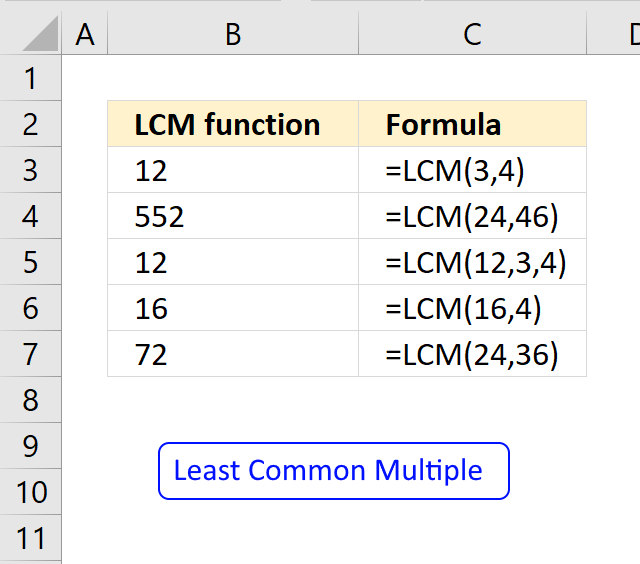Author: Oscar Cronquist Article last updated on April 18, 2018The LCM function calculates the least common multiple. The least common multiple is the smallest positive integer that is a multiple of all integer arguments. Use the LCM function to find fractions with different denominators.

Formula in cell B3:

=LCM(3,4)

### Excel Function Syntax

LCM(number1, [number2], ...)

### Arguments

 number1 Required. The number you want to calculate the least common multiple for. [number2] Optional. Up to 254 additional numbers.